themis contains extra steps for the recipes package for dealing with unbalanced data. The name themis is that of the ancient Greek god who is typically depicted with a balance.

## Installation

You can install the released version of themis from CRAN with:

install.packages("themis")

Install the development version from GitHub with:

# install.packages("pak")
pak::pak("tidymodels/themis")

## Example

Following is a example of using the SMOTE algorithm to deal with unbalanced data

library(recipes)
library(modeldata)
library(themis)

data("credit_data")

credit_data0 <- credit_data %>%
filter(!is.na(Job))

count(credit_data0, Job)
#>         Job    n
#> 1     fixed 2805
#> 2 freelance 1024
#> 3    others  171
#> 4   partime  452

ds_rec <- recipe(Job ~ Time + Age + Expenses, data = credit_data0) %>%
step_impute_mean(all_predictors()) %>%
step_smote(Job, over_ratio = 0.25) %>%
prep()

ds_rec %>%
bake(new_data = NULL) %>%
count(Job)
#> # A tibble: 4 × 2
#>   Job           n
#>   <fct>     <int>
#> 1 fixed      2805
#> 2 freelance  1024
#> 3 others      701
#> 4 partime     701

## Methods

Below is some unbalanced data. Used for examples latter.

example_data <- data.frame(class = letters[rep(1:5, 1:5 * 10)],
x = rnorm(150))

library(ggplot2)

example_data %>%
ggplot(aes(class)) +
geom_bar()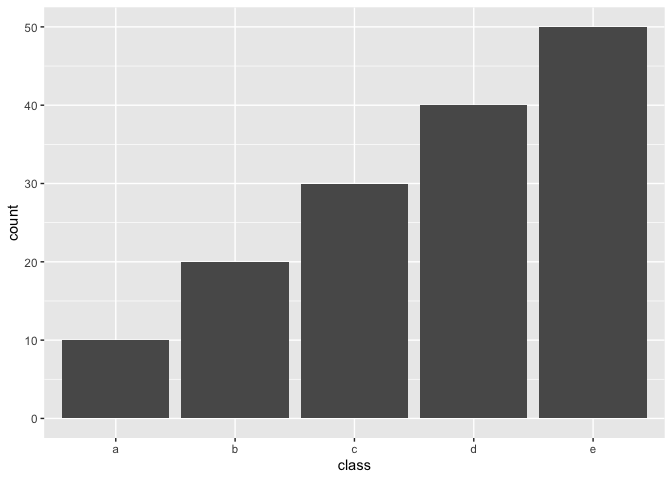### Upsample / Over-sampling

The following methods all share the tuning parameter over_ratio, which is the ratio of the majority-to-minority frequencies.

name function Multi-class
Random minority over-sampling with replacement step_upsample() ✔️
Synthetic Minority Over-sampling Technique step_smote() ✔️
Borderline SMOTE-1 step_bsmote(method = 1) ✔️
Borderline SMOTE-2 step_bsmote(method = 2) ✔️
Adaptive synthetic sampling approach for imbalanced learning step_adasyn() ✔️
Generation of synthetic data by Randomly Over Sampling Examples step_rose()

By setting over_ratio = 1 you bring the number of samples of all minority classes equal to 100% of the majority class.

recipe(~., example_data) %>%
step_upsample(class, over_ratio = 1) %>%
prep() %>%
bake(new_data = NULL) %>%
ggplot(aes(class)) +
geom_bar()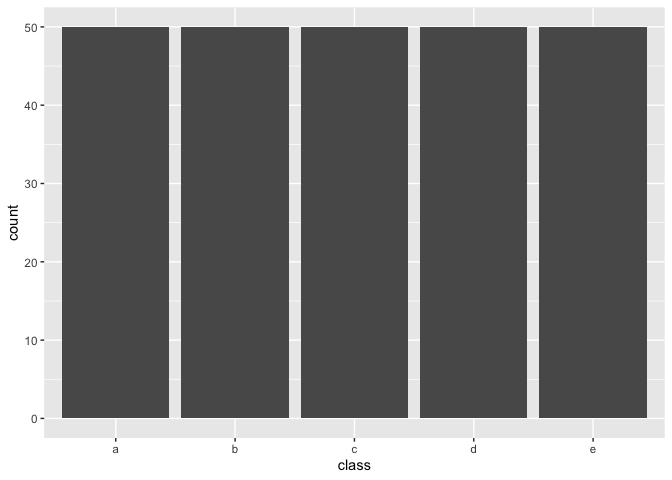and by setting over_ratio = 0.5 we upsample any minority class with less samples then 50% of the majority up to have 50% of the majority.

recipe(~., example_data) %>%
step_upsample(class, over_ratio = 0.5) %>%
prep() %>%
bake(new_data = NULL) %>%
ggplot(aes(class)) +
geom_bar()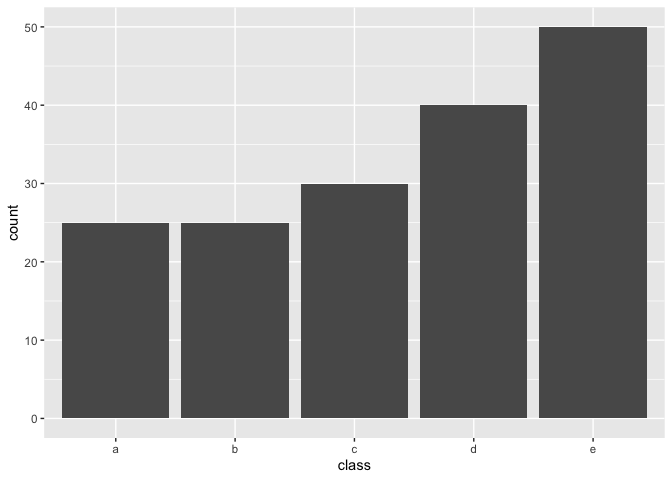### Downsample / Under-sampling

Most of the the following methods all share the tuning parameter under_ratio, which is the ratio of the minority-to-majority frequencies.

name function Multi-class under_ratio
Random majority under-sampling with replacement step_downsample() ✔️ ✔️
NearMiss-1 step_nearmiss() ✔️ ✔️
Extraction of majority-minority Tomek links step_tomek()

By setting under_ratio = 1 you bring the number of samples of all majority classes equal to 100% of the minority class.

recipe(~., example_data) %>%
step_downsample(class, under_ratio = 1) %>%
prep() %>%
bake(new_data = NULL) %>%
ggplot(aes(class)) +
geom_bar()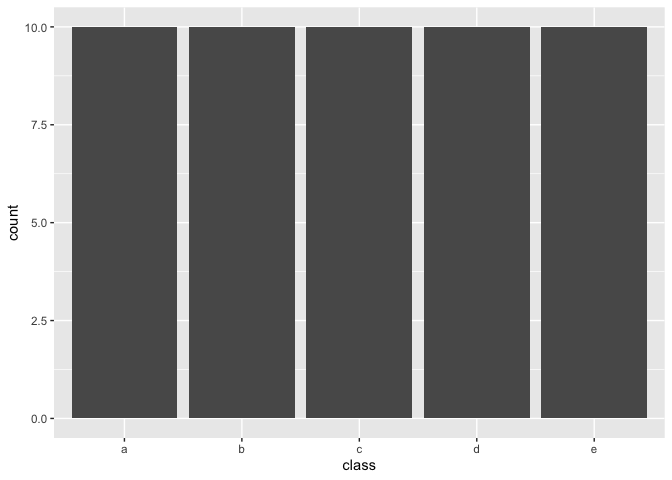and by setting under_ratio = 2 we downsample any majority class with more then 200% samples of the minority class down to have to 200% samples of the minority.

recipe(~., example_data) %>%
step_downsample(class, under_ratio = 2) %>%
prep() %>%
bake(new_data = NULL) %>%
ggplot(aes(class)) +
geom_bar()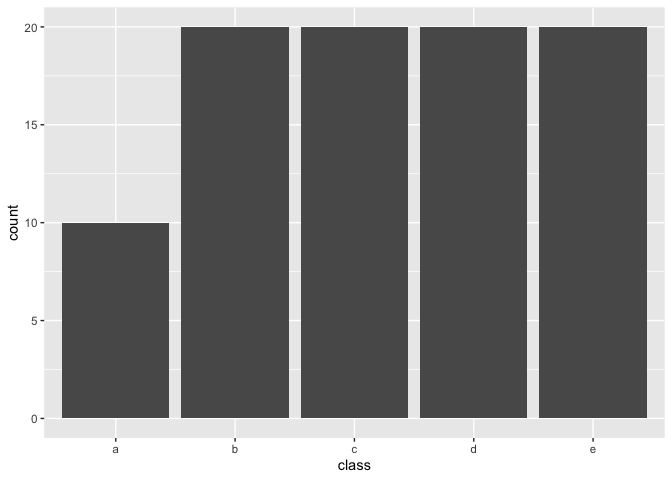## Contributing

This project is released with a Contributor Code of Conduct. By contributing to this project, you agree to abide by its terms.